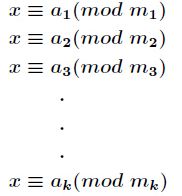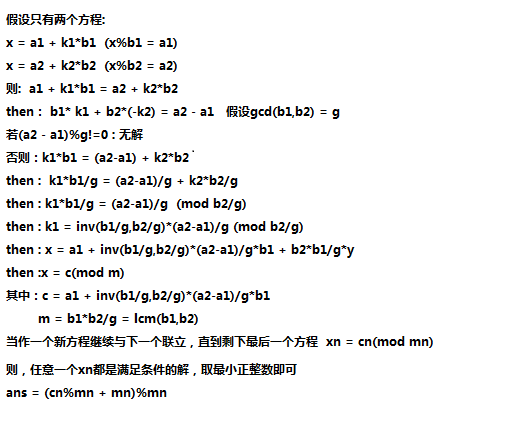## ACM数论----中国剩余定理与拓展中国剩余定理_阿阿阿安的博客-程序员资料

1.假设 n1 % 3 = 2 , n2 % 5 = 3 , n3 % 7 = 2 ;那么如果要使, (n1 + n2) % 3 = 2,那么就要求 n2 也是 3 的倍数 ， 那么如果要使, (n1 + n3) % 3 = 2,那么就要求 n3 也是 3 的倍数 ， 那么如果要使, (n1 + n2 + n3) % 3 = 2,那么就要求 n2和n3 都是 3 的倍数，这是在n1的角度上说的，于是有为了使 （n1 + n2 + n3）成为这样的数字，就要：

（1）（n1 + n2 + n3）%3 = 2成立：n2 和 n3 是 3 的倍数。

（2）（n1 + n2 + n3）%5 = 3成立：n1 和 n3 是5的倍数。

（3）（n1 + n2 + n3）%7 = 2成立：n1 和 n2 是7的倍数。

（1）n1 % 3 =2 并且 n1 是 5 和 7 的公倍数

（2）n2 % 5 = 3并且 n2 是 3 和 7 的公倍数

（3）n3 % 7 = 2并且 n3 是 3 和 5 的公倍数## 因为m1 , m2 , m3 , .....mk是互质的 ， 所以最小公倍数M = m1 * m2 * m3 * .....*mk

x = （a1*M1*inv（M1） + a2 * M2 * inv（M2） + .... + ai * Mi * inv（Mi） + ... + ak*Mk*inv（Mk））%M;

``````#include <iostream>
#include <cstdio>
#include<cstring>
#include<string>
using namespace std;
typedef long long LL;
const int maxn = 100000 + 10;
LL a[maxn],m[maxn],n;
LL ex_gcd(LL a,LL b,LL &x,LL &y){//拓展欧几里得
if(b==0){x = 1;y = 0;return a;}
LL ans = ex_gcd(b,a%b,x,y);
LL temp = x;
x = y;
y = temp - a/b*y;
return ans;
}
LL inv(LL a,LL b){//求逆元
LL x,y;
LL ans = ex_gcd(a,b,x,y);
if(ans!=1)return -1;
if(x<0)x = (x%b + b)%b;
return x;
}
LL China(){//中国剩余定理
LL M = 1;
for(int i = 0;i<n;i++){
M*=m[i];
}
LL sum = 0;
for(int i = 0;i<n;i++){
LL res = M/m[i];
sum = (sum + a[i]*res*inv(res,m[i]))%M;
}
return sum;
}
int main()
{
while(scanf("%lld",&n)!=EOF){
for(int i = 0;i<n;i++){
scanf("%lld%lld",&m[i],&a[i]);
}
LL ans = China();
printf("%lld\n",ans);
}
return 0;
}
``````

poj 1006 Biorhythms

x = n1 + k1*p1

x = n2 + k2*p2

x = n3 + k3*p3

x%p1 = n1%p1即 x % p1 = a1

x%p2 = n2%p2即x % p2 = a2

x%p3 = n3%p3即x % p3 = a3

``````#include <iostream>
#include<cstdio>
#include<string>
#include<cstring>
#include<algorithm>
using namespace std;
int m,a;
int M;
int ex_gcd(int a,int b,int &x,int &y){//拓展欧几里得
if(b==0){x = 1;y = 0;return a;}
int res = ex_gcd(b,a%b,x,y);
int temp = x;
x = y;
y = temp - a/b*y;
return res;
}
int inv(int a,int b){//求逆元
int x,y;
int ans = ex_gcd(a,b,x,y);
if(ans!=1)return -1;
if(x<0)x = (x%b + b)%b;
return x;
}
int China(){//中国剩余定理
int sum = 0;
for(int j = 0;j<3;j++){
int res = M/m[j];
sum = (sum + a[j]*res*inv(res,m[j]))%M;
}
return sum;
}
int main()
{
int initial;
int t = 0;
m = 23;m = 28;m = 33;
M = 1;
for(int i = 0;i<3;i++)M*=m[i];//公倍数
while(scanf("%d%d%d%d",&a,&a,&a,&initial)!=EOF){
t++;
if(a==-1&&a==-1&&a==-1&&initial==-1)break;
for(int i = 0;i<3;i++)a[i]%=m[i];//求a[i]
int ans = China();
if(ans<=initial)ans+=21252;
printf("Case %d: the next triple peak occurs in %d days.\n",t,ans-initial);
}
return 0;
}
``````

（1）上述解决是在模数两两互素的条件下进行的，那么现在如果不两两互素呢？（2）代码：（引入快速乘）

``````#include <iostream>
#include<bits/stdc++.h>
using namespace std;
typedef long long LL;
const int maxn = 100000 + 7;
LL C[maxn],M[maxn];
int n;
LL gcd(LL a,LL b){
return b==0?a:gcd(b,a%b);
}
LL ex_gcd(LL a,LL b,LL &x,LL &y){
if(b==0){x = 1;y = 0;return a;}
LL g = ex_gcd(b,a%b,x,y);
LL temp = x;
x = y;
y = temp - a/b*y;
return g;
}
LL inv(LL a,LL mod){
LL X,Y;
LL g = ex_gcd(a,mod,X,Y);
if(g!=1)return -1;
return (X%mod + mod)%mod;
}
LL mul(LL a,LL b,LL mod){//快速乘法
LL ans = 0;
//cout<<a<<" "<<b<<endl;
while(b){
if(b&1)ans = (ans%mod + a%mod)%mod;
b>>=1;
a = (a%mod + a%mod)%mod;
}
return ans;
}
int main()
{
while(scanf("%d",&n)!=EOF&&n){
for(int i = 0;i<n;i++){
scanf("%lld%lld",&M[i],&C[i]);
}
bool flag = true;
for(int i = 1;i<n;i++){
LL M1 = M[i-1],M2 = M[i],C1 = C[i-1],C2 = C[i];
LL g = gcd(M1,M2);
if((C2-C1)%g){flag = false;break;}
M[i] = M1/g*M2;
LL INV = inv(M1/g,M2/g);
if(INV==-1){flag = false;break;}
C[i] =  C1 + (INV*((C2-C1)/g))%(M2/g)*M1;
C[i] = (C[i]%M[i] + M[i])%M[i];
}
if(!flag)printf("No Solution!\n");
else printf("%lld\n",C[n-1]);
}
return 0;
}
``````

（3）水题 HDU1573

注意 ： 余数为零（整除的坑点！）

``````#include <iostream>
#include<bits/stdc++.h>
using namespace std;
typedef long long LL;
const int maxn = 20+ 7;
LL M[maxn],C[maxn],n,m;
LL gcd(LL a,LL b){
return b==0?a:gcd(b,a%b);
}
LL ex_gcd(LL a,LL b,LL &x,LL &y){
if(b==0){x = 1;y = 0;return a;}
LL g = ex_gcd(b,a%b,x,y);
LL temp = x;
x = y;
y = temp - a/b*y;
return g;
}
LL inv(LL a,LL mod){
LL X,Y;
LL g = ex_gcd(a,mod,X,Y);
if(g!=1)return -1;
return (X%mod + mod)%mod;
}
int main()
{
int T;
scanf("%d",&T);
while(T--){
scanf("%lld%lld",&n,&m);
for(int i = 0;i<m;i++)scanf("%lld",&M[i]);
for(int i = 0;i<m;i++){scanf("%lld",&C[i]);C[i]%=M[i];}
bool flag = true;
for(int i = 1;i<m;i++){
LL M1 = M[i-1],M2 = M[i],C1 = C[i-1],C2 = C[i];
LL g = gcd(M1,M2);
if((C2 - C1)%g){flag = false;break;}
M[i] = M1/g*M2;
LL INV = inv(M1/g,M2/g);
if(INV==-1){flag = false;break;}
C[i] = C1 + INV*(((C2-C1)/g)%(M2/g))*M1;
C[i] = (C[i]%M[i] + M[i])%M[i];
}
if(!flag||C[m-1]>n){printf("0\n");continue;}
LL ans = (n - C[m-1])/M[m-1] + 1;
if(C[m-1]==0)ans--;//x = k*M[m-1],求正整数，不包括0！！
printf("%lld\n",ans);
}
return 0;
}
``````

### js图片预加载实现！_weixin_30265103的博客-程序员资料

var myImage = (function(){　　var imgNode = document.createElement( 'img' );　　document.body.appendChild( imgNode );　　　　return {　　　　　　setSrc: function( src ){　　　　　　　imgNode.src = src;　　　　　　...

### ptoday_19_11_03_WhyDon’tWeKnowHowtoProtectOurTime_lietobrain的博客-程序员资料

Why Don’t We Know How to Protect Our Time?The cognitive errors that make us waste our most valuable resource整合自己想法的总结有时候不可避免需要和啰嗦的邻居互动，需要自己多花额外的时间去回复邮件、忙工作上大大小小的事，开不完的不同会议，把时间很平均的浪费在我们认为“合理”以上这...

### NFS介绍 NFS服务端安装配置 NFS挂载配置选项 exportfs命令 NFS客户端问题_xou6363的博客-程序员资料

1、NFS介绍NFS服务全称是NetWork File System：网络文件系统，最早有sun公司开发的，4.0版本由Netapp公司开发，是基于RPC远程过程调用（Remote Procedure Call）协议的服务应用场景：A,B,C三台机器上需要被访问到的文件是一样的，A共享数据出来，B和C分别取挂载A共享的数据目录，从而B和C访问到的数据和A上的一致。NFS原理图服务端需要启动一个NF...